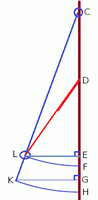# Need generalized equation for Bahama Shutter stay-arm length

#### Cubist

##### Full Member
I'm not sure about the actual question, so before attempting an answer can I ask if this is correct...Red line DL is like a piston so it can change length from $$d_1$$ when fully compressed to $$d_2$$ when fully extended (but with some overlap remaining within the piston for stability). These two lengths are known.

Distance LE needs to be 18". (Or would you rather specify the actual window opening KG=18"?)

Now, given the above, do you want to calculate the position of the fixing points D and L for the piston such that the piston uses its full range? (These fixing positions correspond to lengths CD and CL )